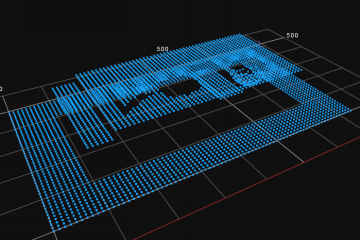# Surface

### Description

The Surface data type represents a cloud of points in a three-dimensional space. The represented point cloud is organized in a precisely defined way. The X coordinate values of the points create an arithmetic progression, and the same goes for the Y coordinate values. Therefore the whole Surface object has a grid structure, which enables to spare a lot of memory in the point cloud representation. It also allows to define regions of interest for a Surface object.A sample Surface object.The same Surface object seen from above. The grid structure is clearly visible.

## Memory Representation

The aforementioned grid structure is described by four values: offsets and scales on X and Y axes, named: XOffset, XScale, YOffset, YScale. The values denotes that the cloud points in the first row of the grid have the Y coordinate equal to YOffset, in the second row the Y coordinate is: YOffset + YScale, in the third row it is: YOffset + 2 * YScale and so on. Similarly, the points in the consecutive columns of the grid have the X coordinate equal to XOffset, XOffset + XScale, XOffset + 2 * XScale and so on.

The Z coordinate values are stored in the same way as the values in an Image object. Just as in there, the Surface values are represented in a one of 6 possible primitive types: sint8, uint8, sint16, uint16, sint32 and real. To obtain the resulting value of the Z coordinate, the value stored has to be multiplied by ZScale and ZOffset has to be added. For every point in the cloud however it is possible that it does not exist. In this case a special value of Z is stored to mark that the point is missing.

## Invalid points

Some surface generation and transformation filters can introduce invalid points, that is, points that have an infinite value in the Z coordinate. An example of such filter could be ArrangePoint3DArray which will insert an invalid point in all locations for which the Z coordinate could not be computed (e.g. due to lack of nearby points in the input array).

Invalid points can be localized using SurfaceValidPointsRegion or replaced with a constant value with a filter called ReplaceInvalidSurfacePoints.

## Remarks

• Single Surface object can allocate no more than 2GB of memory.
• Maximum Surface single dimension should not exceed 65535 (because regions use 16-bit integers for performance reasons).

## Library Definition

Methods:

• int Width() - returns number of grid columns
• int Height() - returns number of grid rows
• int Pitch() - returns byte-distance between consecutive rows
• PlainType Type() - returns type used for representing Z values
• double XOffset() - returns X axis offset
• double XScale() - returns X axis step
• double YOffset() - returns Y axis offset
• double YScale() - returns Y axis step
• double ZOffset() - returns Z axis offset
• double ZScale() - returns Z axis step
• double X( int xIndex ) - returns X coordinate of points in the xIndex column
• double Y( int yIndex ) - returns Y coordinate of points in the yIndex row
• double Z( int xIndex, int yIndex ) - returns Z coordinate of the point defined by given indices
• void* Data() - returns pointer to point cloud data buffer
• T* RowBegin<T>( int i ) - returns pointer to first point in grid row
• T* RowEnd<T>( int i ) - returns pointer to first after last point in grid row
• T* Ptr<T>( int x, int y ) - returns pointer to point at specified coordinates
• T& Value<T>( int i ) - allows to access Z coordinate value at specified coordinates
• T Infinity<T>() - returns the value used for representing a missing point